xPower factorEncyclopedia
The power factor of an AC
Alternating current
In alternating current the movement of electric charge periodically reverses direction. In direct current , the flow of electric charge is only in one direction....

electric power system is defined as the ratio
Ratio
In mathematics, a ratio is a relationship between two numbers of the same kind , usually expressed as "a to b" or a:b, sometimes expressed arithmetically as a dimensionless quotient of the two which explicitly indicates how many times the first number contains the second In mathematics, a ratio is...

of the real power flowing to the load over the apparent power in the circuit, and is a dimensionless number between 0 and 1 (frequently expressed as a percentage, e.g. 0.5 pf = 50% pf). Real power
Power (physics)
In physics, power is the rate at which energy is transferred, used, or transformed. For example, the rate at which a light bulb transforms electrical energy into heat and light is measured in watts—the more wattage, the more power, or equivalently the more electrical energy is used per unit...

is the capacity of the circuit for performing work in a particular time. Apparent power is the product of the current and voltage of the circuit. Due to energy stored in the load and returned to the source, or due to a non-linear load that distorts the wave shape of the current drawn from the source, the apparent power will be greater than the real power.

In an electric power system, a load with a low power factor draws more current than a load with a high power factor for the same amount of useful power transferred. The higher currents increase the energy lost in the distribution system, and require larger wires and other equipment. Because of the costs of larger equipment and wasted energy, electrical utilities will usually charge a higher cost to industrial or commercial customers where there is a low power factor.

Linear loads with low power factor (such as induction motor
Induction motor
An induction or asynchronous motor is a type of AC motor where power is supplied to the rotor by means of electromagnetic induction. These motors are widely used in industrial drives, particularly polyphase induction motors, because they are robust and have no brushes...

s) can be corrected with a passive network of capacitor
Capacitor
A capacitor is a passive two-terminal electrical component used to store energy in an electric field. The forms of practical capacitors vary widely, but all contain at least two electrical conductors separated by a dielectric ; for example, one common construction consists of metal foils separated...

s or inductor
Inductor
An inductor is a passive two-terminal electrical component used to store energy in a magnetic field. An inductor's ability to store magnetic energy is measured by its inductance, in units of henries...

s. Non-linear loads, such as rectifier
Rectifier
A rectifier is an electrical device that converts alternating current , which periodically reverses direction, to direct current , which flows in only one direction. The process is known as rectification...

s, distort the current drawn from the system. In such cases, active or passive power factor correction may be used to counteract the distortion and raise the power factor. The devices for correction of the power factor may be at a central substation, spread out over a distribution system, or built into power-consuming equipment.

## Power factor in linear circuits

In a purely resistive AC circuit, voltage and current waveforms are in step (or in phase), changing polarity at the same instant in each cycle. All the power entering the load is consumed. Where reactive loads are present, such as with capacitor
Capacitor
A capacitor is a passive two-terminal electrical component used to store energy in an electric field. The forms of practical capacitors vary widely, but all contain at least two electrical conductors separated by a dielectric ; for example, one common construction consists of metal foils separated...

s or inductor
Inductor
An inductor is a passive two-terminal electrical component used to store energy in a magnetic field. An inductor's ability to store magnetic energy is measured by its inductance, in units of henries...

s, energy storage in the loads result in a time difference between the current and voltage waveforms. During each cycle of the AC voltage, extra energy, in addition to any energy consumed in the load, is temporarily stored in the load in electric
Electric field
In physics, an electric field surrounds electrically charged particles and time-varying magnetic fields. The electric field depicts the force exerted on other electrically charged objects by the electrically charged particle the field is surrounding...

or magnetic field
Magnetic field
A magnetic field is a mathematical description of the magnetic influence of electric currents and magnetic materials. The magnetic field at any given point is specified by both a direction and a magnitude ; as such it is a vector field.Technically, a magnetic field is a pseudo vector;...

s, and then returned to the power grid a fraction of a second later in the cycle. The "ebb and flow" of this nonproductive power increases the current in the line. Thus, a circuit with a low power factor will use higher currents to transfer a given quantity of real power than a circuit with a high power factor. A linear load does not change the shape of the waveform of the current, but may change the relative timing (phase) between voltage and current.

Circuits containing purely resistive heating elements (filament lamps, strip heaters, cooking stoves, etc.) have a power factor of 1.0. Circuits containing inductive or capacitive elements (electric motors, solenoid
Solenoid
A solenoid is a coil wound into a tightly packed helix. In physics, the term solenoid refers to a long, thin loop of wire, often wrapped around a metallic core, which produces a magnetic field when an electric current is passed through it. Solenoids are important because they can create...

valves, lamp ballasts
Electrical ballast
An electrical ballast is a device intended to limit the amount of current in an electric circuit. A familiar and widely used example is the inductive ballast used in fluorescent lamps, to limit the current through the tube, which would otherwise rise to destructive levels due to the tube's...

, and others ) often have a power factor below 1.0.

### Definition and calculation

AC power flow has the three components: real power (also known as active power) (P), measured in watt
Watt
The watt is a derived unit of power in the International System of Units , named after the Scottish engineer James Watt . The unit, defined as one joule per second, measures the rate of energy conversion.-Definition:...

s (W); apparent power (S), measured in volt-ampere
Volt-ampere
A volt-ampere is the unit used for the apparent power in an electrical circuit, equal to the product of root-mean-square voltage and RMS current. In direct current circuits, this product is equal to the real power in watts...

s (VA); and reactive power (Q), measured in reactive volt-amperes (var).

The power factor is defined as: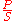.

In the case of a perfectly sinusoidal
Sine wave
The sine wave or sinusoid is a mathematical function that describes a smooth repetitive oscillation. It occurs often in pure mathematics, as well as physics, signal processing, electrical engineering and many other fields...

waveform, P, Q and S can be expressed as vectors that form a vector triangle such that: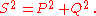If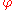is the phase angle
Phase angle
In the context of vectors and phasors, the term phase angle refers to the angular component of the polar coordinate representation. The notation A\ang \!\ \theta,   for a vector with magnitude A and phase angle θ, is called angle notation.In the context of periodic phenomena, such as a wave,...

between the current and voltage, then the power factor is equal to the cosine of the angle,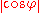, and: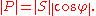Since the units are consistent, the power factor is by definition a dimensionless number between 0 and 1. When power factor is equal to 0, the energy flow is entirely reactive, and stored energy in the load returns to the source on each cycle. When the power factor is 1, all the energy supplied by the source is consumed by the load. Power factors are usually stated as "leading" or "lagging" to show the sign of the phase angle.

If a purely resistive load is connected to a power supply, current and voltage will change polarity in step, the power factor will be unity (1), and the electrical energy flows in a single direction across the network in each cycle. Inductive loads such as transformers and motors (any type of wound coil) consume reactive power with current waveform lagging the voltage. Capacitive loads such as capacitor banks or buried cable generate reactive power with current phase leading the voltage. Both types of loads will absorb energy during part of the AC cycle, which is stored in the device's magnetic or electric field, only to return this energy back to the source during the rest of the cycle.

For example, to get 1 kW of real power, if the power factor is unity, 1 kVA of apparent power needs to be transferred (1 kW ÷ 1 = 1 kVA). At low values of power factor, more apparent power needs to be transferred to get the same real power. To get 1 kW of real power at 0.2 power factor, 5 kVA of apparent power needs to be transferred (1 kW ÷ 0.2 = 5 kVA). This apparent power must be produced and transmitted to the load in the conventional fashion, and is subject to the usual distributed losses in the production and transmission processes.

Electrical loads consuming alternating current power
AC power
Power in an electric circuit is the rate of flow of energy past a given point of the circuit. In alternating current circuits, energy storage elements such as inductance and capacitance may result in periodic reversals of the direction of energy flow...

consume both real power and reactive power. The vector sum of real and reactive power is the apparent power. The presence of reactive power causes the real power to be less than the apparent power, and so, the electric load has a power factor of less than 1.

### Power factor correction of linear loads

A high power factor is generally desirable in a transmission system to reduce transmission losses and improve voltage regulation at the load. It is often desirable to adjust the power factor of a system to near 1.0. When reactive elements supply or absorb reactive power near the load, the apparent power is reduced. Power factor correction may be applied by an electrical power transmission utility to improve the stability and efficiency of the transmission network. Individual electrical customers who are charged by their utility for low power factor may install correction equipment to reduce those costs.

Power factor correction brings the power factor of an AC power circuit closer to 1 by supplying reactive power of opposite sign, adding capacitors or inductors that act to cancel the inductive or capacitive effects of the load, respectively. For example, the inductive effect of motor loads may be offset by locally connected capacitors. If a load had a capacitive value, inductors (also known as reactors in this context) are connected to correct the power factor. In the electricity industry, inductors are said to consume reactive power and capacitors are said to supply it, even though the reactive power is actually just moving back and forth on each AC cycle.

The reactive elements can create voltage fluctuations and harmonic noise when switched on or off. They will supply or sink reactive power regardless of whether there is a corresponding load operating nearby, increasing the system's no-load losses. In the worst case, reactive elements can interact with the system and with each other to create resonant conditions, resulting in system instability and severe overvoltage
Overvoltage
When the voltage in a circuit or part of it is raised above its upper design limit, this is known as overvoltage. The conditions may be hazardous...

fluctuations. As such, reactive elements cannot simply be applied without engineering analysis.
An automatic power factor correction unit consists of a number of capacitor
Capacitor
A capacitor is a passive two-terminal electrical component used to store energy in an electric field. The forms of practical capacitors vary widely, but all contain at least two electrical conductors separated by a dielectric ; for example, one common construction consists of metal foils separated...

s that are switched by means of contactor
Contactor
A contactor is an electrically controlled switch used for switching a power circuit, similar to a relay except with higher current ratings. A contactor is controlled by a circuit which has a much lower power level than the switched circuit....

s. These contactors are controlled by a regulator that measures power factor in an electrical network. Depending on the load and power factor of the network, the power factor controller will switch the necessary blocks of capacitors in steps to make sure the power factor stays above a selected value.

Instead of using a set of switched capacitor
Capacitor
A capacitor is a passive two-terminal electrical component used to store energy in an electric field. The forms of practical capacitors vary widely, but all contain at least two electrical conductors separated by a dielectric ; for example, one common construction consists of metal foils separated...

Synchronous motor
A synchronous electric motor is an AC motor distinguished by a rotor spinning with coils passing magnets at the same rate as the power supply frequency and resulting rotating magnetic field which drives it....

can supply reactive power. The reactive power drawn by the synchronous motor is a function of its field excitation. This is referred to as a synchronous condenser
Synchronous condenser
In electrical engineering, a synchronous condenser is adevice identical to a synchronous motor, whose shaft is not connected to anything but spins freely. Its purpose is not to convert electric power to mechanical power or vice versa, but to adjust conditions on the electric power transmission grid...

. It is started and connected to the electrical network
Electrical network
An electrical network is an interconnection of electrical elements such as resistors, inductors, capacitors, transmission lines, voltage sources, current sources and switches. An electrical circuit is a special type of network, one that has a closed loop giving a return path for the current...

. It operates at a leading power factor and puts vars onto the network as required to support a system’s voltage
Voltage
Voltage, otherwise known as electrical potential difference or electric tension is the difference in electric potential between two points — or the difference in electric potential energy per unit charge between two points...

or to maintain the system power factor at a specified level.

The condenser’s installation and operation are identical to large electric motor
Electric motor
An electric motor converts electrical energy into mechanical energy.Most electric motors operate through the interaction of magnetic fields and current-carrying conductors to generate force...

s. Its principal advantage is the ease with which the amount of correction can be adjusted; it behaves like an electrically variable capacitor. Unlike capacitors, the amount of reactive power supplied is proportional to voltage, not the square of voltage; this improves voltage stability on large networks. Synchronous condensors are often used in connection with high voltage direct current transmission projects or in large industrial plants such as steel mill
Steel mill
A steel mill or steelworks is an industrial plant for the manufacture of steel.Steel is an alloy of iron and carbon. It is produced in a two-stage process. First, iron ore is reduced or smelted with coke and limestone in a blast furnace, producing molten iron which is either cast into pig iron or...

s.

A non-linear load on a power system is typically a rectifier (such as used in a power supply), or some kind of arc discharge device such as a fluorescent lamp
Fluorescent lamp
A fluorescent lamp or fluorescent tube is a gas-discharge lamp that uses electricity to excite mercury vapor. The excited mercury atoms produce short-wave ultraviolet light that then causes a phosphor to fluoresce, producing visible light. A fluorescent lamp converts electrical power into useful...

, electric welding
Welding
Welding is a fabrication or sculptural process that joins materials, usually metals or thermoplastics, by causing coalescence. This is often done by melting the workpieces and adding a filler material to form a pool of molten material that cools to become a strong joint, with pressure sometimes...

machine, or arc furnace. Because current in these systems is interrupted by a switching action, the current contains frequency components that are multiples of the power system frequency. Distortion power factor is a measure of how much the harmonic distortion of a load current decreases the average power transferred to the load.

### Non-sinusoidal components

Non-linear loads change the shape of the current waveform from a sine wave
Sine wave
The sine wave or sinusoid is a mathematical function that describes a smooth repetitive oscillation. It occurs often in pure mathematics, as well as physics, signal processing, electrical engineering and many other fields...

to some other form. Non-linear loads create harmonic
Harmonic
A harmonic of a wave is a component frequency of the signal that is an integer multiple of the fundamental frequency, i.e. if the fundamental frequency is f, the harmonics have frequencies 2f, 3f, 4f, . . . etc. The harmonics have the property that they are all periodic at the fundamental...

currents in addition to the original (fundamental frequency) AC current. Filters consisting of linear capacitors and inductors can prevent harmonic currents from entering the supplying system.

In linear circuits having only sinusoidal currents and voltages of one frequency, the power factor arises only from the difference in phase between the current and voltage. This is "displacement power factor". The concept can be generalized to a total, distortion, or true power factor where the apparent power includes all harmonic components. This is of importance in practical power systems that contain non-linear loads such as rectifiers, some forms of electric lighting, electric arc furnace
Electric arc furnace
An electric arc furnace is a furnace that heats charged material by means of an electric arc.Arc furnaces range in size from small units of approximately one ton capacity up to about 400 ton units used for secondary steelmaking...

s, welding equipment, switched-mode power supplies
Switched-mode power supply
A switched-mode power supply is an electronic power supply that incorporates a switching regulator in order to be highly efficient in the conversion of electrical power...

and other devices.

A typical multimeter
Multimeter
A multimeter or a multitester, also known as a VOM , is an electronic measuring instrument that combines several measurement functions in one unit. A typical multimeter may include features such as the ability to measure voltage, current and resistance...

will give incorrect results when attempting to measure the AC current drawn by a non-sinusoidal load; the instruments sense the average value of a rectified waveform. The average response is then calibrated to the effective, RMS
Root mean square
In mathematics, the root mean square , also known as the quadratic mean, is a statistical measure of the magnitude of a varying quantity. It is especially useful when variates are positive and negative, e.g., sinusoids...

value. An RMS sensing multimeter must be used to measure the actual RMS currents and voltages (and therefore apparent power). To measure the real power or reactive power, a watt meter designed to work properly with non-sinusoidal currents must be used.

### Distortion power factor

The distortion power factor describes how the harmonic distortion of a load current decreases the average power transferred to the load.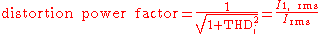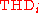is the total harmonic distortion
Total harmonic distortion
The total harmonic distortion, or THD, of a signal is a measurement of the harmonic distortion present and is defined as the ratio of the sum of the powers of all harmonic components to the power of the fundamental frequency...

of the load current. This definition assumes that the voltage stays undistorted (sinusoidal, without harmonics). This simplification is often a good approximation in practice.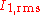is the fundamental component of the current and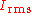is the total current - both are root mean square
Root mean square
In mathematics, the root mean square , also known as the quadratic mean, is a statistical measure of the magnitude of a varying quantity. It is especially useful when variates are positive and negative, e.g., sinusoids...

-values.

The result when multiplied with the displacement power factor (DPF) is the overall, true power factor or just power factor (PF):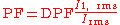### Switched-mode power supplies

A particularly important class of non-linear loads is the millions of personal computers that typically incorporate switched-mode power supplies
Switched-mode power supply
A switched-mode power supply is an electronic power supply that incorporates a switching regulator in order to be highly efficient in the conversion of electrical power...

(SMPS) with rated output power ranging from a few watts to more than 1 kW. Historically, these very-low-cost power supplies incorporated a simple full-wave rectifier that conducted only when the mains instantaneous voltage exceeded the voltage on the input capacitors. This leads to very high ratios of peak-to-average input current, which also lead to a low distortion power factor and potentially serious phase and neutral loading concerns.

A typical switched-mode power supply
Switched-mode power supply
A switched-mode power supply is an electronic power supply that incorporates a switching regulator in order to be highly efficient in the conversion of electrical power...

first makes a DC bus, using a bridge rectifier or similar circuit. The output voltage is then derived from this DC bus. The problem with this is that the rectifier
Rectifier
A rectifier is an electrical device that converts alternating current , which periodically reverses direction, to direct current , which flows in only one direction. The process is known as rectification...

is a non-linear device, so the input current is highly non-linear. That means that the input current has energy at harmonic
Harmonic
A harmonic of a wave is a component frequency of the signal that is an integer multiple of the fundamental frequency, i.e. if the fundamental frequency is f, the harmonics have frequencies 2f, 3f, 4f, . . . etc. The harmonics have the property that they are all periodic at the fundamental...

s of the frequency of the voltage.

This presents a particular problem for the power companies, because they cannot compensate for the harmonic current by adding simple capacitors or inductors, as they could for the reactive power drawn by a linear load. Many jurisdictions are beginning to legally require power factor correction for all power supplies above a certain power level.

Regulatory agencies such as the EU have set harmonic limits as a method of improving power factor. Declining component cost has hastened implementation of two different methods. To comply with current EU standard EN61000-3-2, all switched-mode power supplies
Switched-mode power supply
A switched-mode power supply is an electronic power supply that incorporates a switching regulator in order to be highly efficient in the conversion of electrical power...

with output power more than 75 W must include passive PFC, at least. 80 PLUS
80 PLUS
80 PLUS is an initiative to promote energy efficiency in computer power supply units . It certifies products that have more than 80% energy efficiency at 20%, 50% and 100% of rated load, and a power factor of 0.9 or greater at 100% load....

power supply certification requires a power factor of 0.9 or more.

#### Passive PFC

The simplest way to control the harmonic
Harmonic
A harmonic of a wave is a component frequency of the signal that is an integer multiple of the fundamental frequency, i.e. if the fundamental frequency is f, the harmonics have frequencies 2f, 3f, 4f, . . . etc. The harmonics have the property that they are all periodic at the fundamental...

current is to use a filter
Electronic filter
Electronic filters are electronic circuits which perform signal processing functions, specifically to remove unwanted frequency components from the signal, to enhance wanted ones, or both...

: it is possible to design a filter that passes current only at line frequency (e.g. 50 or 60 Hz). This filter reduces the harmonic current, which means that the non-linear device now looks like a linear
Linear
In mathematics, a linear map or function f is a function which satisfies the following two properties:* Additivity : f = f + f...

load. At this point the power factor can be brought to near unity, using capacitors or inductors as required. This filter requires large-value high-current inductors, however, which are bulky and expensive.

A passive PFC requires an inductor larger than the inductor in an active PFC, but costs less.

This is a simple way of correcting the nonlinearity of a load by using capacitor banks. It is not as effective as active PFC.

Passive PFCs are typically more power efficient than active PFCs. Efficiency is not to be confused with the PFC, though many computer hardware reviews conflate them. A passive PFC on a switching computer PSU has a typical power efficiency of around 96%, while an active PFC has a typical efficiency of about 94%.

#### Active PFC

An "active power factor corrector" (active PFC) is a power electronic
Power electronics
Power electronics is the application of solid-state electronics for the control and conversion of electric power.-Introduction:Power electronic converters can be found wherever there is a need to modify a form of electrical energy...

system that controls the amount of power drawn by a load in order to obtain a power factor as close as possible to unity. In most applications, the active PFC controls the input current of the load so that the current waveform is proportional to the mains voltage waveform (a sine wave). The purpose of making the power factor as close to unity (1) as possible is to make the load circuitry that is power factor corrected appear purely resistive (apparent power equal to real power). In this case, the voltage and current are in phase and the reactive power consumption is zero. This enables the most efficient delivery of electrical power from the power company to the consumer.
Some types of active PFC are:
• Boost
Boost converter
A boost converter is a power converter with an output DC voltage greater than its input DC voltage. It is a class of switching-mode power supply containing at least two semiconductor switches and at least one energy storage element...

• Buck
Buck converter
A buck converter is a step-down DC to DC converter. Its design is similar to the step-up boost converter, and like the boost converter it is a switched-mode power supply that uses two switches , an inductor and a capacitor....

• Buck-boost
Buck-boost converter
The buck–boost converter is a type of DC-to-DC converter that has an output voltage magnitude that is either greater than or less than the input voltage magnitude.Two different topologies are called buck–boost converter....

Active power factor correctors can be single-stage or multi-stage.

In the case of a switched-mode power supply, a boost converter
Boost converter
A boost converter is a power converter with an output DC voltage greater than its input DC voltage. It is a class of switching-mode power supply containing at least two semiconductor switches and at least one energy storage element...

is inserted between the bridge rectifier and the main input capacitors. The boost converter attempts to maintain a constant DC bus voltage on its output while drawing a current that is always in phase with and at the same frequency as the line voltage. Another switchmode converter inside the power supply produces the desired output voltage from the DC bus. This approach requires additional semiconductor switches and control electronics, but permits cheaper and smaller passive components. It is frequently used in practice. For example, SMPS
Switched-mode power supply
A switched-mode power supply is an electronic power supply that incorporates a switching regulator in order to be highly efficient in the conversion of electrical power...

with passive PFC can achieve power factor of about 0.7–0.75, SMPS with active PFC, up to 0.99 power factor, while a SMPS without any power factor correction has a power factor of only about 0.55–0.65.

Due to their very wide input voltage range, many power supplies with active PFC can automatically adjust to operate on AC power from about 100 V (Japan) to 230 V (Europe). That feature is particularly welcome in power supplies for laptops.

## Importance of power factor in distribution systems

The significance of power factor lies in the fact that utility companies supply customers with volt-ampere
Volt-ampere
A volt-ampere is the unit used for the apparent power in an electrical circuit, equal to the product of root-mean-square voltage and RMS current. In direct current circuits, this product is equal to the real power in watts...

s, but bill them for watt
Watt
The watt is a derived unit of power in the International System of Units , named after the Scottish engineer James Watt . The unit, defined as one joule per second, measures the rate of energy conversion.-Definition:...

s. Power factors below 1.0
require a utility to generate more than the minimum volt-amperes necessary to supply the real power (watts). This increases generation and transmission costs. For example, if the load power factor were as low as 0.7, the apparent power would be 1.4 times the real power used by the load. Line current in the circuit would also be 1.4 times the current required at 1.0 power factor, so the losses in the circuit would be doubled (since they are proportional to the square of the current). Alternatively all components of the system such as generators, conductors, transformers, and switchgear would be increased in size (and cost) to carry the extra current.

Utilities typically charge additional costs to customers who have a power factor below some limit, which is typically 0.9 to 0.95. Engineers are often interested in the power factor of a load as one of the factors that affect the efficiency of power transmission.

With the rising cost of energy and concerns over the efficient delivery of power, active PFC has become more common in consumer electronics. Current Energy Star
Energy Star
Energy Star is an international standard for energy efficient consumer products originated in the United States of America. It was first created as a United States government program during the early 1990s, but Australia, Canada, Japan, New Zealand, Taiwan and the European Union have also adopted...

guidelines for computers (ENERGY STAR Program Requirements for Computers Version 5.0) call for a power factor of ≥ 0.9 at 100% of rated output in the PC's power supply
Power supply unit (computer)
A power supply unit converts mains AC to low-voltage regulated DC power for the internal components of the computer. Modern personal computers universally use a switched-mode power supply...

. According to a white paper authored by Intel and the U.S. Environmental Protection Agency‎, PCs with internal power supplies will require the use of active power factor correction to meet the ENERGY STAR 5.0 Program Requirements for Computers.

In Europe, IEC 555-2 requires power factor correction be incorporated into consumer products.

## Measuring power factor

Power factor in a single-phase circuit (or balanced three-phase circuit) can be measured with the wattmeter-ammeter-voltmeter method, where the power in watts is divided by the product of measured voltage and current. The power factor of a balanced polyphase circuit is the same as that of any phase. The power factor of an unbalanced polyphase circuit is not uniquely defined.

A direct reading power factor meter can be made with a moving coil meter of the electrodynamic type, carrying two perpendicular coils on the moving part of the instrument. The field of the instrument is energized by the circuit current flow. The two moving coils, A and B, are connected in parallel with the circuit load. One coil, A, will be connected through a resistor and the second coil, B, through an inductor, so that the current in coil B is delayed with respect to current in A. At unity power factor, the current in A is in phase with the circuit current, and coil A provides maximum torque, driving the instrument pointer toward the 1.0 mark on the scale. At zero power factor, the current in coil B is in phase with circuit current, and coil B provides torque to drive the pointer towards 0. At intermediate values of power factor, the torques provided by the two coils add and the pointer takes up intermediate positions.

Another electromechanical instrument is the polarized-vane type. In this instrument a stationary field coil produces a rotating magnetic field, just like a polyphase motor. The field coils are connected either directly to polyphase voltage sources or to a phase-shifting reactor if a single-phase application. A second stationary field coil, perpendicular to the voltage coils, carries a current proportional to current in one phase of the circuit. The moving system of the instrument consists of two vanes that are magnetized by the current coil. In operation the moving vanes take up a physical angle equivalent to the electrical angle between the voltage source and the current source. This type of instrument can be made to register for currents in both directions, giving a four-quadrant display of power factor or phase angle.

Digital instruments can be made that either directly measure the time lag between voltage and current waveforms and so calculate the power factor, or by measuring both true and apparent power in the circuit and calculating the quotient. The first method is only accurate if voltage and current are sinusoidal; loads such as rectifiers distort the waveforms from the sinusoidal shape.

## Mnemonics

English-language power engineering students are advised to remember:
"ELI the ICE man" or "ELI on ICE" – the voltage E leads the current I in an inductor L, the current leads the voltage in a capacitor C.

Another common mnemonic is CIVIL – in a capacitor (C) the current (I) leads voltage (V), voltage (V) leads current (I) in an inductor (L).